# Implementation of Wave Function Collapse Algorithm in Houdini for 3D Content Generation

This project is inspired and built on top of the 2D implementation of WFC in Houdini by if. I really appreciate his support through this journey as I started this project with zero Houdini knowledge. You can view his original article here in Chinese.

This project studies Wave Function Collapse (WFC), a constraint-based algorithm for Procedural Content Generation(PDG), and implements it in Houdini, a procedural content creation tool to create a 3D content generator that outputs diverse prototypes which could potentially be used in the design and entertainment industry.

## Wave Function Collapse Algorithm / Model Synthesis Algorithm

Sidenote: Paul Merrell developed Model Synthesis Algorithm in 2007 which generates similar results as WFC. You can see the comparison here and view the source code here.

WFC is an algorithm developed by Maxim Gumin as a texture synthesis method based on simple configuration or sample images. It is a constraint-based procedural algorithm that is inspired and named after the concept wave function collapse from quantum physics. In quantum physics, wave function collapse is the idea that the unobserved state of a particle can be anything. As soon as the particle is observed, the possibilities disappear and the wave function collapses. The same idea is the backbone of the procedural algorithm. WFC can be implemented with two diﬀerent models, the tiled model and the overlapping model. In this report, the focus will entirely be on the tiled model.

The WFC algorithm initializes a grid, where each cell in the grid will be deﬁned as a slot. The WFC algorithm’s tiled model variant occupies each slot in the grid with a module. A module contains information about the 3D model and the constraints for the module’s neighbours. In the complete unobserved state, each slot has the possibility to be filled by every module possible. The modules have lists of possible neighbours they allow next to them in the grid. This means that if one slot is collapsed down to a single possible module, the neighbouring slots will also be restricted in possible modules because of the neighbouring collapsed slot. This constraint of possible modules then spreads to neighbouring slots, also constraining them in possible modules. WFC can be extended to high dimensions but the most common is two and three dimensions. For a two dimensional system, four neighbours are needed for a square grid and for three dimensions six neighbours. WFC can also be used in diﬀerent grid shapes, the square being the most common and the shape used in this report. Hexagon grids with six neighbours are also popular.

1. Create a grid with the dimension of the output, and each slot contains a list of possible modules.
2. Initialize the grid in a completely unobserved state, i.e. with all modules added to all slots’ possibility spaces.
3. Repeat the following steps: Observation: Find the slot with the lowest entropy. Entropy is a measurement of uncertainty and disorder. In general, a slot with high entropy is one with lots of possible tiles remaining in its wavefunction. Which tile it will eventually collapse to is still very uncertain. By contrast, a square with low entropy is one with few possible tiles remaining in its wavefunction. Which tile it will eventually collapse to is already very constrained. In our case, find the slot with the smallest possibility space. If multiple slots are tied, select one of those at random. If all slots only have one module left in their possibility space or all modules only have zero modules left. Break the cycle and go to step 4. Collapse this slot’s possibility space at random down to a single module. This is done by removing all but one module from the possibility space. As they are removed they start a chain reaction that reduces the size of neighbouring possibility spaces. Propagation: propagate the information gained on the previous observation step. The propagation step propagates through the whole grid and checks if changes made in the observation step aﬀect the possibility space of the neighbouring slots. The propagation step is the most expensive part of the algorithm and is recursive and dependent on the size of the grid. In the simplest approach, it propagates through every single slot in the grid each time a change in a possibility space happens
4. By now all the slots possibility space either contains exactly one module or they all contain exactly zero modules. If all slots contain zero modules, the algorithm has hit a contradiction and the result should be discarded. If the slots all contain one module, the algorithm is completed and the result can be returned.

It is perfectly ﬁne to do a pre-collapse of a slot. If a speciﬁc module is needed on a speciﬁc slot because of design considerations or such, this can be done after step 2. Simply remove all other modules from the given slots possibility space and begin the algorithm with the propagation step. The algorithm will then build around the given module and works as if it had been collapsed randomly in step 3. For example, if you don’t want certain modules to appear in certain slots, like edge slots, you can eliminate those modules from the possibility space. Vice versa, you can specify certain modules in certain slots to meet your design constraints.

## Previous Application of Wave Function Collapse

WFC was initially developed by Maxim Gumin for generating bitmaps that are locally similar to the input bitmap.(See Fig.1)Fig. 1 Example of 2D procedural art using the overlapping model by Maxim Gumin

The algorithm was later adapted for other scenarios such as 3D procedural art and game level generation. Marian Kleineberg created an inﬁnite world in three dimensions using WFC (see Fig. 2). The world can extend inﬁnitely in any direction the player chooses to go in. The tilesets consist of around 100 diﬀerent modules, with custom constraints for each of them.

Another game developer who has contributed to the popularization of Wave Function Collapse is Oskar Stålberg. In his recently released game, Townscaper, Oskar Stålberg applied WFC to generate towns with beautiful tilesets and irregular grids. (see Fig. 3)

## Implementation of WFC in 2D

In order to implement WFC in Houdini, I started with a two-dimensional approach first. It is easier to test out the workflow and also can be extended to three dimensions for the next steps. This workflow is basically a translation from if’s article.

Step 1: Find 2D tiles sample — Wang Tiles

Wang tiles were first proposed by mathematician Hao Wang in 1961. A set of square tiles, with each tile edge of a fixed color, are arranged side by side in a rectangular grid. All four edges of each tile must ‘match’ (have the same color as) their adjoining neighbor. With careful tile design, a complete array can produce a large image without visual ‘breaks’ between tiles. This helps computer game designers create large tiled backgrounds from a small set of tile images.

Here is a set of Wang tiles. You can see that every tile has two different types of edge; blue or yellow. This gives 2x2x2x2 (written as 2⁴), or 16 possible combinations. Hence the complete set contains 16 different tiles.(see Fig. 4)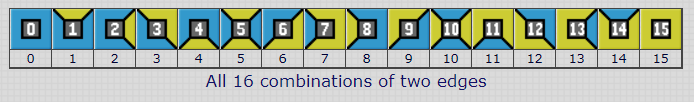Fig.4 A set of Wang Tiles with two different types of edge

Step 2: Import Wang Tiles into Houdini with a Null node

• Create a geometry node at the obj level named “tiles”, this is where we import the Wang Tiles images as tiles for WFC. Inside the “tiles” node:
• Create a COP network, and specify Wang Tiles images file path. In Houdini, A COP network contains compositing nodes (COPs) for manipulating 2D pixel data.
• Create a material network to properly render the images.
• Create an object network, under the network, create a list of geometry nodes that separates UVs into reasonably flat, non-overlapping groups for further usage in the workflow
• Create a list of points that represent the image tiles, and add attributes to save the path of image files, coppath, and soppath.
• Add an attribute called frequency that decides how frequent each tile will appear in the outcome

Using Python to loop through the image directory , import the files, create networks, nodes, and points I described above, and add attributes to each point. (See Code snippet below)

`node = hou.pwd()geo = node.geometry()add_paths = node.node(‘../add_paths’)imgdir = node.parm(‘imagedir’).eval()ext = node.parm(‘ext’).eval()irang = node.parmTuple(‘range’).eval()import oscopnet = node.node(‘../copnet’)copnet.deleteItems(copnet.children())matnet = node.node(‘../matnet’)matnet.deleteItems(matnet.children())objnet = node.node(‘../objnet’)objnet.deleteItems(objnet.children())code = “int pt;\n”for i in range(irang, irang+1): filenode=copnet.createNode(‘file’, ‘img_’+str(i)) fn = os.path.join(imgdir, str(i)+ext) filenode.parm(‘filename1’).set(fn) filenode.moveToGoodPosition()  matnode =matnet.createNode(‘principledshader::2.0’, ‘mat_’+str(i)) matnode.parm(‘basecolor_useTexture’).set(1) matnode.parm(‘basecolorr’).set(1) matnode.parm(‘basecolorg’).set(1) matnode.parm(‘basecolorb’).set(1) matnode.parm(‘basecolor_texture’).set(‘op:’+filenode.path()) matnode.moveToGoodPosition()  geo = objnet.createNode(‘geo’, ‘tile_’+str(i)) grid = geo.createNode(‘grid’) grid.parm(‘sizex’).set(1) grid.parm(‘sizey’).set(1) grid.parm(‘rows’).set(2) grid.parm(‘cols’).set(2) uv = geo.createNode(‘uvunwrap’) uv.parm(‘spacing’).set(0) uv.setInput(0, grid) mat = geo.createNode(‘material’) mat.parm(‘shop_materialpath1’).set(matnode.path()) mat.setInput(0, uv) mat.setDisplayFlag(True) mat.setRenderFlag(True) grid.moveToGoodPosition() uv.moveToGoodPosition() mat.moveToGoodPosition() geo.moveToGoodPosition()  line = “pt = addpoint(0, {0,0,0});\n”; line += “setpointattrib(0, ‘path’, pt, ‘{}’);\n”.format(fn) line += “setpointattrib(0, ‘coppath’, pt, ‘{}’);\n”.format(filenode.path()) line += “setpointattrib(0, ‘soppath’, pt, ‘{}’);\n”.format(geo.path()) code += line add_paths.parm(‘snippet’).set(code)`

After setting up the Null node with the custom parameters and code, click “Execute” to generate copnet, matnet, objnet, and update “add_paths” node.(see Fig.5)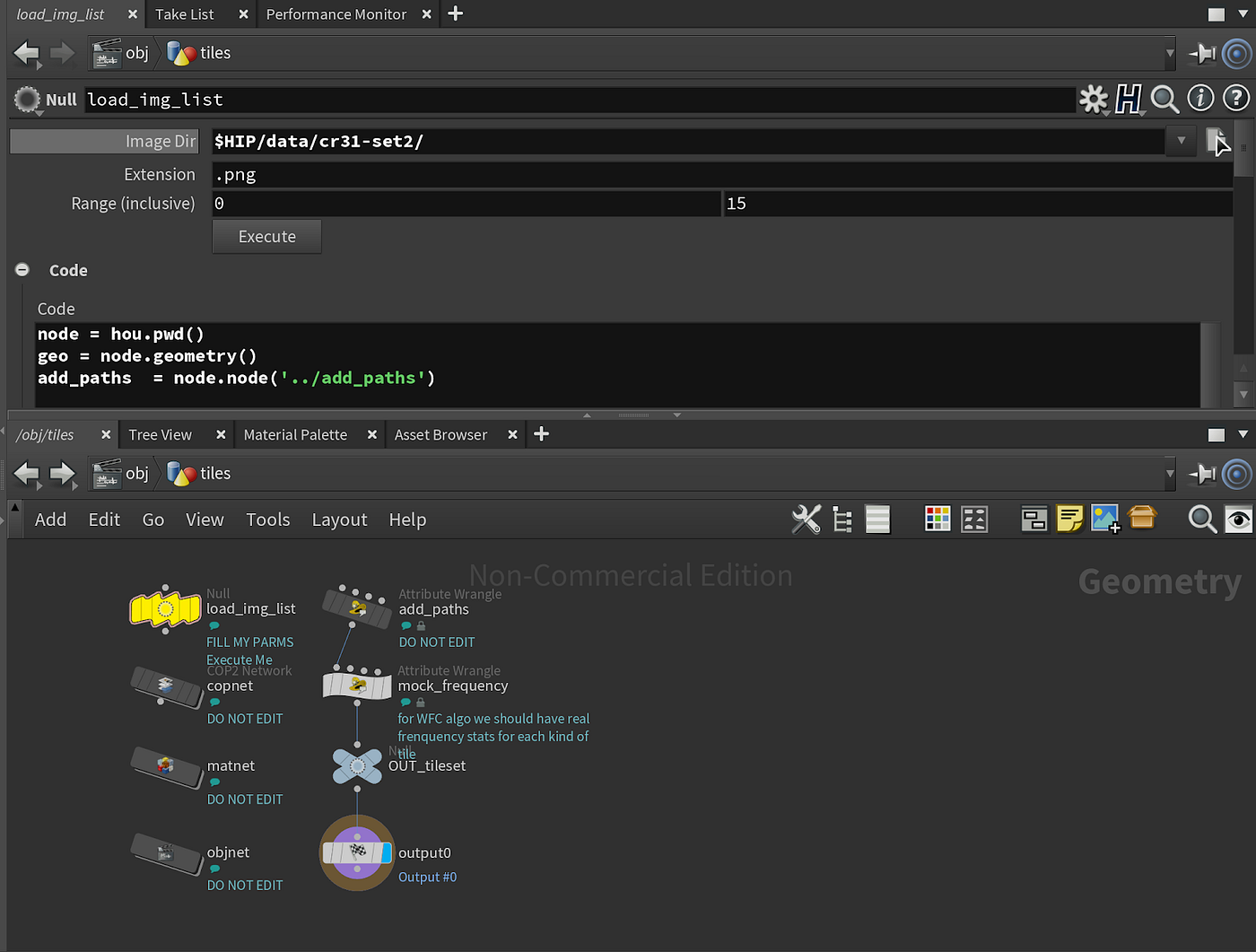Fig.5 Screenshot of “tiles” to import tiles and prepare for WFC

Step 3 Extract color information from four edges of tiles

Create a geometry node named “rules”, side by side with “tiles” node we created earlier. (see Fig. 6)

Inside the “rules” node, in order to decide which tile can go beside which tile, we need to need the feature colors of four edges of each tile. Using “objmerge” node to reference the “tiles” node we created earlier, with a new attribute called “colorPixel” to store the colors of four edges of tiles, a python node is added to extract the pixels of four edges.(see Fig.7) Inside of the Python node, we could use “getPixelByUV” to get pixel values of four edges. (see code snippet)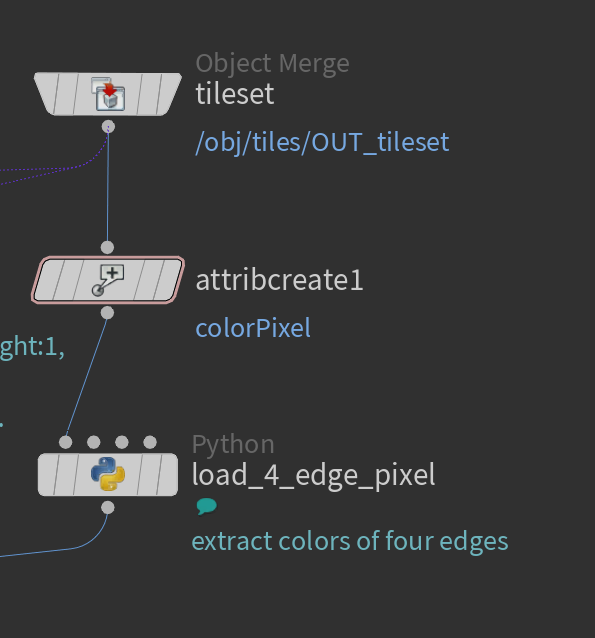Fig. 7 Screenshot of workflow to extract colors of four edges
`import mathnode = hou.pwd()geo = node.geometry()margin = node.parm('margin').eval()def dopt(point):  coppath = point.attribValue('coppath')   copnode = hou.node(coppath)  samplepts = ( (0.5, 1.0-margin), #up                (1.0-margin, 0.5), #right                (0.5, margin), #down                (margin, 0.5) )#left  samplepixels = []  for uv in samplepts:    colorPixel = copnode.getPixelByUV('C', uv, uv)     r,g,b = map(lambda x: math.floor(x*64), colorPixel)    encoded = int(r*64*64+g*64+b)    samplepixels.append(encoded)    #print "{} -> {}\n".format(uv, encoded)      point.setAttribValue('colorPixel', samplepixels)for pt in geo.points():  dopt(pt)`

With the attribute “colorPixel”, we saved the colors of four edges of each tile. (see Fig. 8)

Step 4 Record tiles adjacency rules for WFC

In order to record the rules like if tile A can be placed to the N direction of tile B, we could use a 3D array. If legal[A][B][N] == 1, tile A can be placed to the N direction of tile B. If legal[A][B][N] == 0, tile A can not be placed to the N direction of tile B. Since it is not straightforward to store 3D arrays in SOP in Houdini, we created a N * N * 4 point cloud to store the adjacency rules.(see Fig, 9) After creating the points, we then added an attribute named “legal” to store the adjacency rules. In the Python node, we used the previous stored color info of each tile, and compared the color of edges of tiles, to see if color of edge top matches color of edge bottom, color of edge left matches color of edge right. In this case, point(geo, ‘islegal’, A*N*4 + B*4 + D) is the same as legal[A][B][D]. (see code snippet)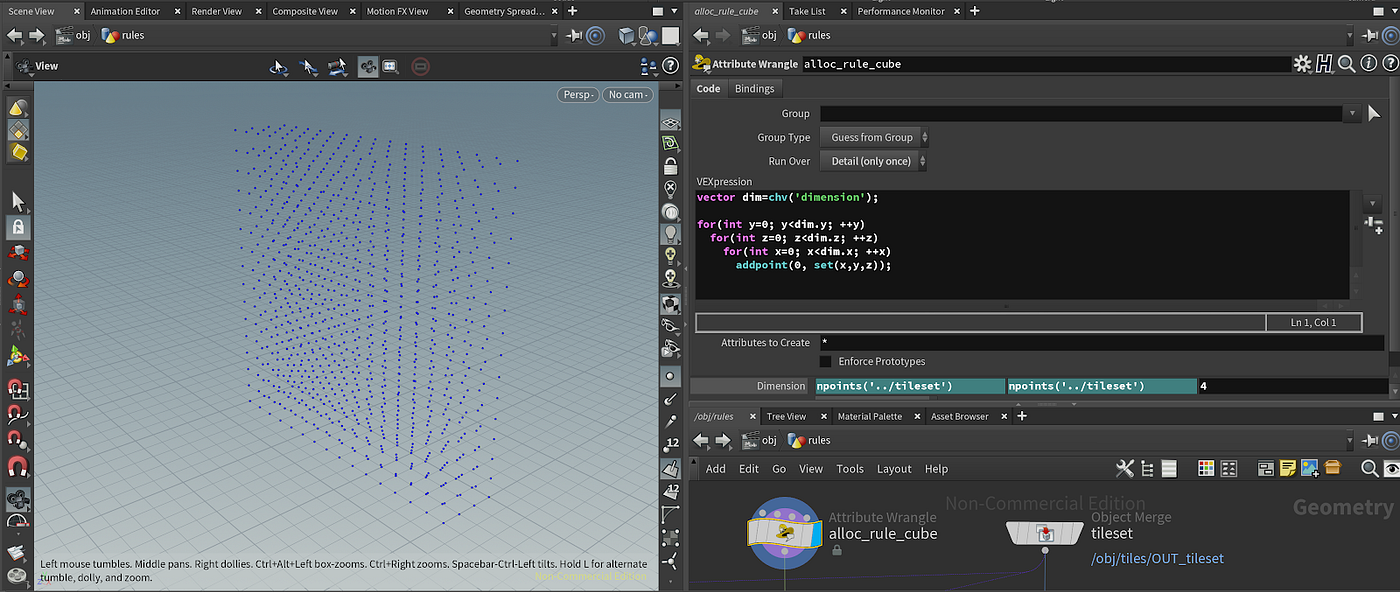Fig. 9 Screenshot of the point cloud N * N * 4
`node = hou.pwd() geo = node.geometry()dimension = map(int,                hou.node('../alloc_rule_cube').               parmTuple('dimension').eval()            )N = dimensionimport numpy as nprules=np.zeros(dimension,dtype=int)#return a new array of given shape and type, filled with zerosgiven_geo = node.inputs().geometry() # load_4_edge_pixel as input, second inputgiven=np.zeros((N, 4),dtype=int)i = 0for pt in given_geo.points():  given[i:]=pt.attribValue('colorPixel')  print(pt.attribValue('colorPixel'))  i+=1for i in range(N):  for j in range(N):    rules[i][j] = given[i] == given[j] ## j above i    rules[i][j] = given[i] == given[j] ## i j    rules[i][j] = given[i] == given[j] ## j below i    rules[i][j] = given[i] == given[j] ## j i    for k in range(4):      geo.point(i*N*4+j*4+k).setAttribValue('islegal', rules[i][j][k])`

After adding adjacency rules, and visualizing the rules, we can view the legal points and illegal points. (see Fig. 10)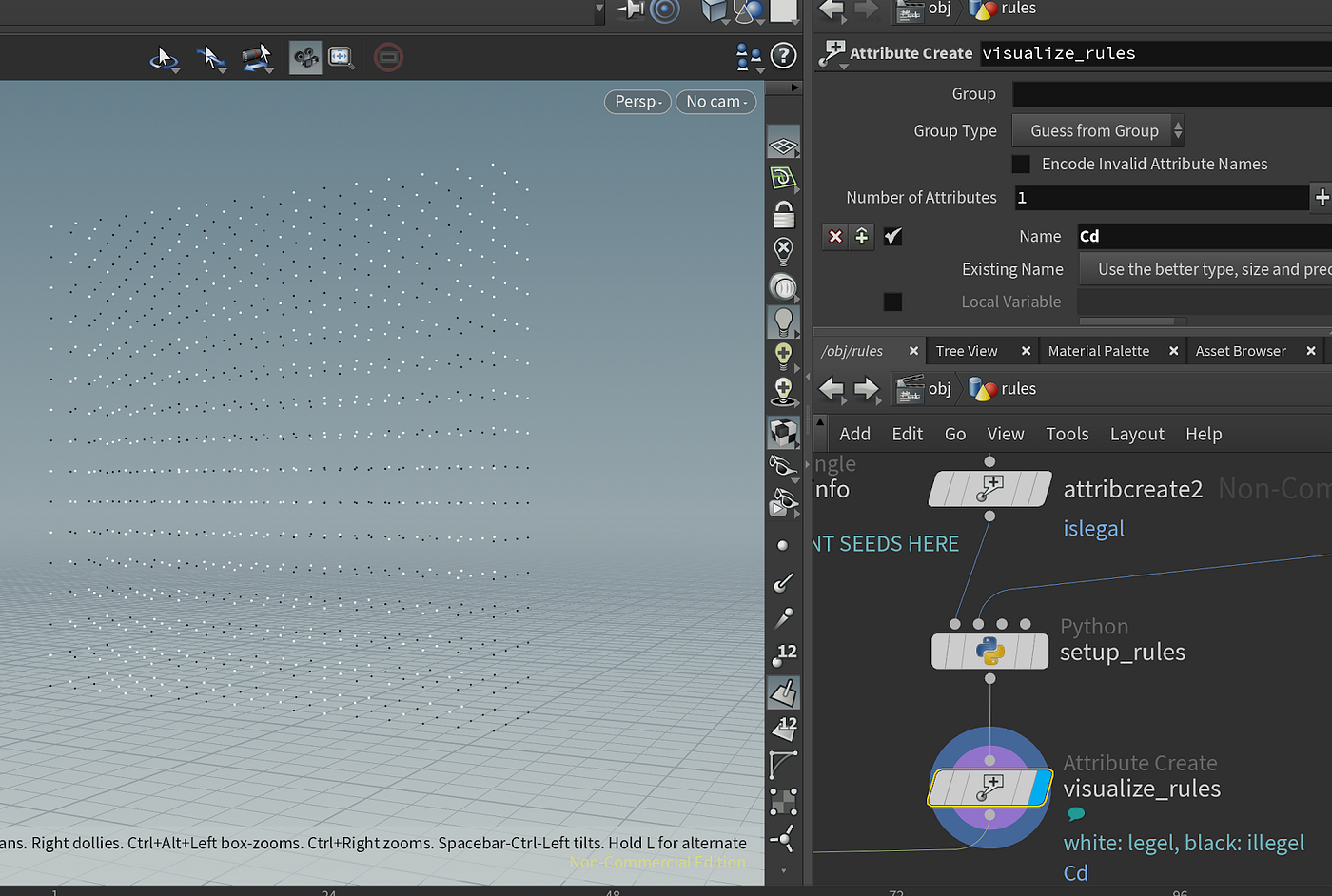Fig. 10 Screenshot to view the “islegal” adjacency rules (White point is 1, Black point is 0)

Step 5 Create an output grid

This is the “Sudoku” board that the user can fill in with tiles. Users can initialize the grid with specified sizes and record the info as the detail attributes.( See Fig. 11)

We initialize the grid in a completely unobserved state, with all modules added to all slots’ possibility spaces.(see Fig. 12)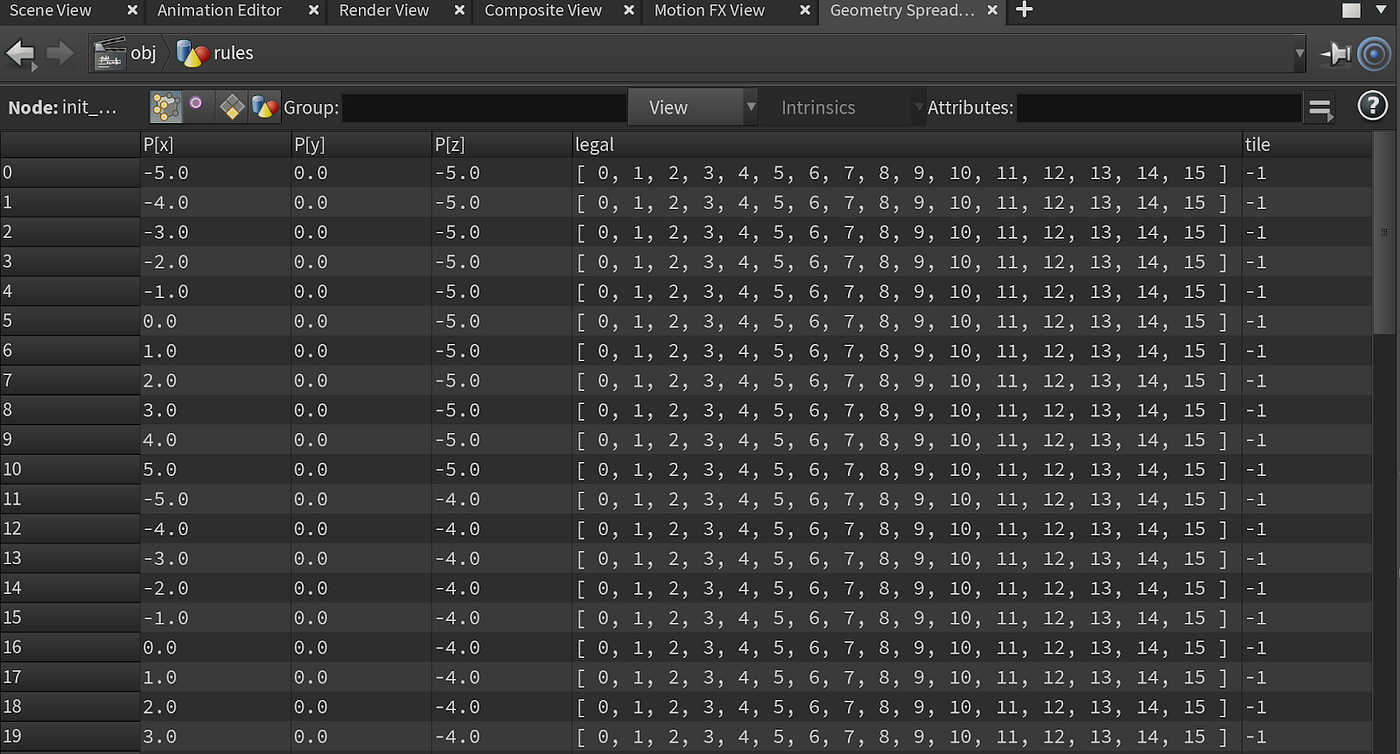Fig. 12 Screenshot of grid points, add all tiles into possibility space(“legal”), “tile” is the final tile that will be chosen, initialized with -1

Step 6 Use solver to apply WFC algorithm for simulation

Normally in geometry networks each frame is self-contained, This node lets you create effects where a surface network modifies the previous frame’s geometry, allowing you to create iterative feedback, automata, and simulation-like effects. First input of solver is the grid, and second input is adjacency rules of the tiles. (see Fig. 13)

Inside of “solver” node, based on the original C# implementation, the algorithm is refactored in Vex. In this case, with the initialized grid, all the grids have the same amount of possible tiles, so pick a random slot to collapse, update the possibility space of other slots, repeat this process until all the slots are collapsed or hit a contradiction, and the process stops.(see Fig. 14)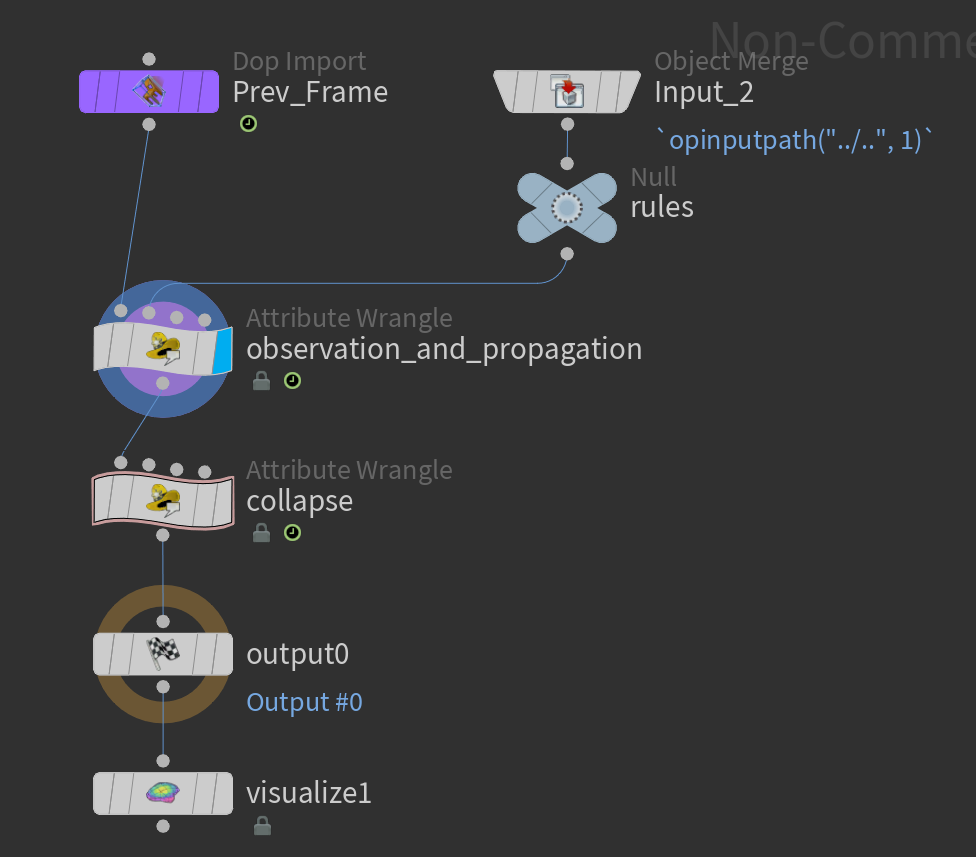Fig .14 Screenshot — inside the “solver” node, integrate WFC with “ observation_and_propagation” and “collapse” in Vex

For the “observation_and_propagation” and “collapse” part, find neighbors in each direction, and remove the impossible tiles from the possibility spaces based on the adjacency rules.(see code snippets)

“observation_and_propagation” code snippet:

`if (!i@giveup) {  int rules[] = {};  resize(rules, npoints(1));  for (int i=0; i<npoints(1); ++i) {    rules[i] = int(point(1, 'islegal', i));  }  vector2 dirs[] = {    {0, -1}, // up    {1, 0},  // right    {0, 1},  // down    {-1,0}   // left  };    int ncollapsed = 0;  for (int i=0; i<i@numpt; ++i) {    int row = i/i@cols;    int col = i%i@cols;    int ptlegal[] = point(0, 'legal', i);    int pttile   = point(0, 'tile', i);    //printf("%i %i \n", ptlegal, pttile);    // found conflict    if (len(ptlegal)==0 && pttile==-1) {      i@giveup = 1;      setpointgroup(0, 'badpt', i, 1);      setpointattrib(0, 'Cd', i, {1,0,0});      break;    } else if (pttile>=0) {      ++ncollapsed;    }    // look around    for (int didx=0; didx<4; ++didx) {      int dcol = int(dirs[didx].x);      int drow = int(dirs[didx].y);      // printf("%i %i \n", dcol, drow);      if (row+drow<0 || row+drow>=i@rows)        continue;      if (col+dcol<0 || col+dcol>=i@cols)        continue;      int neighborpt = i+i@cols*drow + dcol; //?      int neighbor = point(0, 'tile', neighborpt);      if (neighbor>=0) { // determinded        int impossible[]={};        foreach(int candidate; ptlegal) {          if (rules[candidate*i@ntiles*4+neighbor*4+didx] == 0) {            if (find(impossible, candidate)<0) {              append(impossible, candidate);            }          }        }        foreach(int imp; impossible) {          removevalue(ptlegal, imp);        }      }    } // for each direction    setpointattrib(0, 'legal', i, ptlegal);  } // for each point  // finished  if (ncollapsed == npoints(0)) {    i@giveup = 1;  }} // giveup`

“collapse” code snippet:

`int empty[]={};int mostDetermindedPts[] = {};int mostDetermindedCadidateCount = i@ntiles+1;if (!i@giveup) {  int collapsed = 0;  for (int i=0; i<npoints(0); ++i) {    int legal[]=point(0, 'legal', i);    if (len(legal)==1) {      setpointattrib(0, 'tile', i, legal);      setpointattrib(0, 'legal', i, empty);       collapsed = 1;    } else if (len(legal)>1 && len(legal)<mostDetermindedCadidateCount) {      mostDetermindedCadidateCount = len(legal);      mostDetermindedPts = {};      append(mostDetermindedPts, i);    } else if (len(legal)==mostDetermindedCadidateCount) {      append(mostDetermindedPts, i);    }  }  // no determinded tile now  // choose a random tile from most determinded set to collapse  if (!collapsed) {    int luckytileidx = int(floor(rand(f@seed+@Frame*1.3)*len(mostDetermindedPts)));    int luckytile = mostDetermindedPts[luckytileidx];    i[]@mostDetermindedPts = mostDetermindedPts;    i@luckytile = luckytile;    i@luckytileidx = luckytileidx;    // collapse based on frequency stats    int ptlegal[] = point(0, 'legal', luckytile);    float ptfrequency[] = {};    resize(ptfrequency, len(ptlegal));    for (int legalidx=0; legalidx<len(ptlegal); ++legalidx) {      ptfrequency[legalidx] = f[]@frequency[ptlegal[legalidx]];      if (legalidx>0) // accumulate        ptfrequency[legalidx]+=ptfrequency[legalidx-1];    }    float r = rand(f@seed*11.1 + @Frame*0.11) * ptfrequency[-1];    int lucyidx = -1;    for (int idx=0; idx<len(ptlegal); ++idx) {      if (ptfrequency[idx]>=r) {        lucyidx = idx;        break;      }    }    if (lucyidx>=0) {      setpointattrib(0, 'tile', luckytile, ptlegal[lucyidx]);      setpointattrib(0, 'legal', luckytile, empty);      collapsed = 1;    }  }}`

Step 7 Visualization to display the result

After setting up the solver to apply WFC, we need to visualize the outcome of the WFC. The workflow loops through all the grids, and copies the tiles onto the slots. (see Fig. 15)

Now, click the “play” button, and we can see the result of the WFC. (see Fig. 16). If you want to see different results, you can change the “seed” attribute and/or “frequency” attribute to generate different outcomes.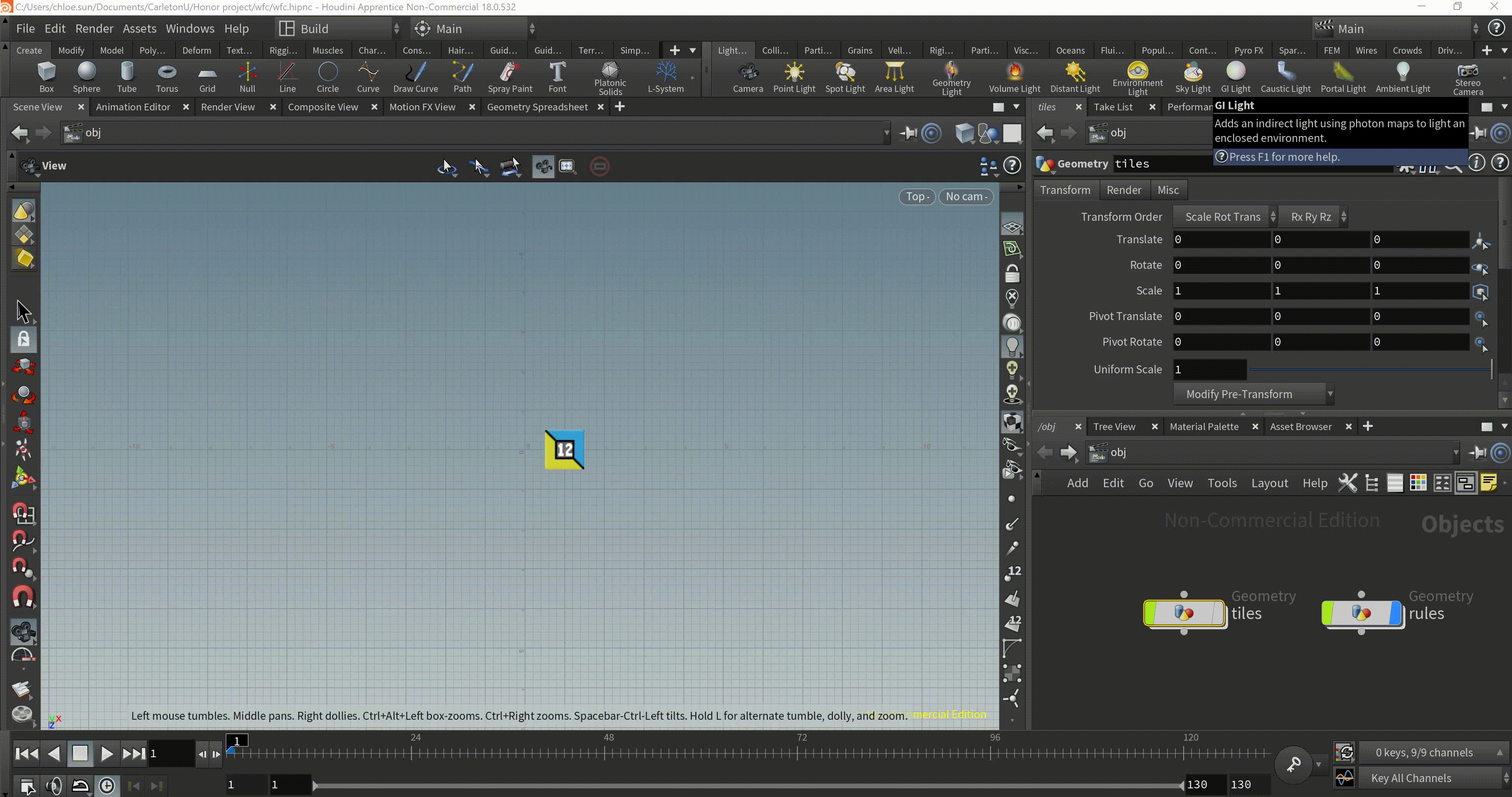Fig. 16 Outcome of using Wang Tiles to generate pattern without visual break

## Implementation of WFC in 3D

Finally,we are here to implement it in 3D. With 2D implementation workflow ready, we can extend the WFC in 3D. We can keep the most workflow and set up from 2D, and address two issues to make it work in 3D.

Issue 1: How to set up the adjacency rules for 3D modules

Issue 2: How to refactor WFC from 4 directions to 6 directions

Step 1 Build 3D modules

In order to test WFC in 3D, I started with very simple modules. I created two modules and after the rotations, there are 15 modules altogether. (see Fig. 17)

Step 2 Set up the adjacency rules for modules

Instead of using the color match to decide which module can go beside which in the 2D implementation, we could use cut area match for the 3D modules. I wrapped each module with an invisible bounding box, and measured the areas of module surface and bounding box surfaces if they intersect. For each module, we added the “cut_areas” as an attribute for assigning adjacency rules in the next step.(see code snippet and Fig. 18)

`float cut_areas[] ={};string soppath = opfullpath(s@soppath);string path = concat("op:",soppath);cut_areas = detail(path, 'cut_areas');f[]@cut_areas = cut_areas;`

Step 3 Refactor the “setup_rules” node to assign adjacent rules

We could compare the cut areas of two surfaces of two modules to decide if they can be placed together. Instead of thinking four directions, we need to consider six directions. (see code snippet)

`node = hou.pwd() geo = node.geometry()dimension = map               hou.node('../alloc_rule_cube').               parmTuple('dimension').eval()            ) print(dimension) N = dimensionimport numpy as nprules=np.zeros(dimension,dtype=int)given_geo = node.inputs().geometry()given=np.zeros((N, 6),dtype=int)i = 0for pt in given_geo.points():  cut_areas = map(float, pt.floatListAttribValue('cut_areas'))  given = given.astype(float)   given[i:] = cut_areas        i+=1print(given)for i in range(N):  for j in range(N):    rules[i][j] = (given[i] == given[j] )    rules[i][j] = (given[i] == given[j] )     rules[i][j] = (given[i] == given[j] )    rules[i][j] = (given[i] == given[j] )    rules[i][j] = (given[i] == given[j] )    rules[i][j] = (given[i] == given[j] )         for k in range(6):      geo.point(i+j*N*6+k*N).setAttribValue('islegal', rules[i][j][k])`

Step 4 Refactor solver node for WFC logic

Similarly, inside the solver node, for the “observation_and_propagation” node, we need to add another dimension, from (x, y) to (x, y, z). (see code snippet)

`if (!i@giveup) {  int rules[] = {};  resize(rules, npoints(1));  for (int i=0; i<npoints(1); ++i) {    rules[i] = int(point(1, 'islegal', i)); // 0 or 1  }  vector dirs[] = {    {0, 0, -1}, // up 0    {1, 0, 0},  // right 1    {0, 0, 1},  // down 2    {-1, 0, 0}, // left 3    {0, -1, 0}, // bottom 4    {0, 1, 0}   // top 5  };  int ncollapsed = 0;  for (int i=0; i<i@numpt; ++i) {    int px =  i % i@cols;    int py =  i / (i@cols*i@rows);    int pz =  (i-px-i@cols*i@rows*py)/i@cols;     int cor[] = array(px, py, pz);     int ptlegal[] = point(0, 'legal', i);     int pttile   = point(0, 'tile', i);     // found conflict    if (len(ptlegal)==0 && pttile==-1) {      i@giveup = 1;      setpointgroup(0, 'badpt', i, 1);      setpointattrib(0, 'Cd', i, {1,0,0});      break;    } else if (pttile>=0) {      ++ncollapsed;    }    // look around    // find the neighbor point number in each direction    for (int didx=0; didx<6; ++didx) {      int dx = int(dirs[didx].x);      int dy = int(dirs[didx].y);      int dz = int(dirs[didx].z);      if (px+dx<0 || px+dx>=i@cols)        continue;      if (py+dy<0 || py+dy>=i@height)        continue;      if (pz+dz<0 || pz+dz>=i@rows)        continue;            int neighborpt = (dx+px) + i@cols*i@rows*(dy+py) + i@cols*(dz+pz); //neighbor point number in certain direction      int pair[] = array(i, neighborpt);      //printf("%i \n", pair);      int neighbor = point(0, 'tile', neighborpt);      // if neighbor's tile is determined      if (neighbor>=0) { // determinded        int impossible[]={}; // impossible tiles        foreach(int candidate; ptlegal) {          if (rules[candidate+i@ntiles*6*neighbor+i@ntiles*didx] == 0) { // [tileA][tileB][direction]            if (find(impossible, candidate)<0) { //Finds an item in an array or string.return negative number if not found              append(impossible, candidate);            }          }        }        foreach(int imp; impossible) {          removevalue(ptlegal, imp);        }      }    } // for each direction    setpointattrib(0, 'legal', i, ptlegal);  } // for each point  // finished  if (ncollapsed == npoints(0)) {    i@giveup = 1;  }} // giveup`

Step 5 Run and play

After refactoring the workflow and code, we can click play and see the outcome in 3D with 15 tube modules (see Fig. 19)

## Conclusion

This report attempted to explore a constraint based PCG algorithm WFC. I studied the algorithm from its source code, researched the application of WFC in video games, and I learned Houdini from scratch and implemented WFC in 2D and extended it to 3D. In order to achieve an ideal result, the users need to have a functional module design in mind and test the design with the workflow back and forth, and it is easy to reach contradictions. The future effort could be implementing the back tracking feature to undo certain steps when users end up in a deadend. Overall, WFC could be a useful tool in Houdini for designers and developers to create diverse and meaningful content in 2D and 3D.

## Bibliography

 Tobias Nordvig Møller, Jonas Aksel Billeskov, Expanding Wave Function Collapse with Growing Grids for Procedural Content Generation, Page 9–11, 2019

 Maxim Gumin. Wave function collapse. https://github.com/mxgmn/WaveFunctionCollapse, 2016.

 Marian Kleineberg. marian42wavefunctioncollapse. https://github.com/marian42/wavefunctioncollapse, 2018.

 Oskar Stålberg, Townscaper, https://store.steampowered.com/app/1291340/Townscaper/

 Maxim Gumin, Simple tiled model https://github.com/mxgmn/WaveFunctionCollapse/blob/master/SimpleTiledModel.cs

--

--